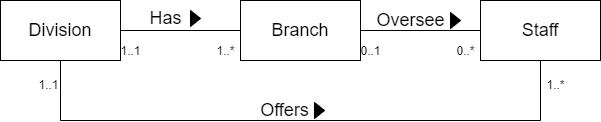# 数据库系统（7）：ER模型

ER模型是一种自上而下 ( $top-down$ ) 的数据库设计方法，该方法首先确定那些被称为实体的重要数据和这些数据之间的联系，实体和联系时ER模式中必备的元素。然后添加更多的细节信息，如属性、约束等。
统一建模语言 ( $Unified\ \ Modeling\ \ Language$, $UML$ ) 是软件工程项目的工业标准建模语言，我们使用UML的符号集来绘制ER模型。

## 1 实体类型

实体类型 ( $Entity\ \ types$ ) 是被企事业单位认可的、能够独立存在的一组具有相同属性的对象，是ER模型的基本概念。实体类型既可以是物理 ( 真实 ) 存在的，也可以是概念 ( 抽象 ) 存在的对象。实体出现 ( $entity\ \ occurrence$ ) 是实体类型中可唯一标识的一个对象。在没有歧义的时候，实体类型和实体出现都可以直接简称为实体。
在UML中，实体类型通过一个标有名字的矩形标识，每个实体名字的首字母是大写的。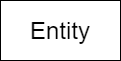## 2. 联系类型

联系类型 ( $Relationship\ \ types$ ) 是实体类型间一组有意义的关联。每个联系类型都被赋予一个能够描述其功能的名字。联系出现 ( $Relationship\ \ occurrence$ ) 是由参与该联系的各个实体类型的一个出现组成的可被唯一标识的关联。
在UML中，每个联系类型都表现为用线将相关的实体类型联系起来，并在线上标上联系的名字，通常使用一个动词或者动词短语命名。同样的，首字母也应该大写。一个联系还要标识方向，意味着这个联系仅在一个方向上有意义。可以在名字旁边添加一个箭头符号，如 $\blacktriangleleft$ 来标识方向。当我们要表示 $A\ \ Has\ \ B$ ( $A$，$B$ 为实体，$Has$ 为联系类型 ) 时可以用如下方法：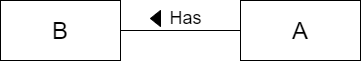### 2.1 联系类型的度

联系类型的 ( $degree$ ) 是指参与联系的实体类型的个数。包含在某个联系类型中的实体被看作参与者 ( $participants$ )，参与者的数目就是度。度为 $2$ 的联系称为二元联系。上例中的 $Has$ 就是一个典型的二元 ( $binary$ ) 联系，该联系的参与者是 $A$ 和 $B$ 。同理，度为 $3$ 的联系称为三元 ( $ternary$ ) 联系。度大于 $2$ 的联系称为复杂联系。
在复杂联系中，联系使用一个菱形符号表示，箭头可以省略。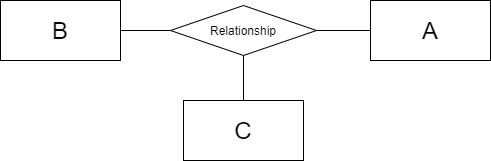### 2.2 递归联系

递归联系 ( $Recursive\ \ relationship$ ) 是指同一个实体类型以不同的角色多次参与了同一个联系类型。递归联系有时也叫做一元 ( $unary$ ) 联系。角色名称 ( $Role\ \ names$ ) 可以添加在联系上，用于表示每个实体类型在联系中的意义。
实体 $Staff$ 表示公司员工，一个主管负责监视员工的同时，他也是公司员工，因此该主管需要自己“监视”自己。第一次参与时的角色名称是 $Supervisor$ ，第二次参与时的角色名称是 $Supervisee$ 。如下所示：角色名称并不限于递归联系。如果两个实体间存在多余一种联系时也可以使用角色名称。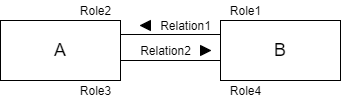## 3. 属性

属性是实体或联系类型所具有的某一特性。属性域 ( $Attribute\ \ domain$ ) 是单个属性或者多个属性所允许的取值集合。多个属性可以共享一个域。属性可以分为：简单 ( $simple$ ) 属性和组合 ( $composite$ ) 属性；单值 ( $single-valued$ ) 属性和多值 ( $multi-valued$ ) 属性；导出 ( $derived$ ) 属性。

### 3.1 简单属性和组合属性

简单属性是指由独立存在的单个部分组成的属性，不能再被划分为更小的部分。简单属性有时也可以称为原子 ( $atomic$ ) 属性。组合属性是指由多个部分组成的属性，每个部分都可以单独存在。

### 3.2 单值属性和多值属性

单值属性是在实体类型的每个实例出现中都只取一个单值的属性。多值属性是在实体类型的某些实例出现中可能取多个值的属性。

### 3.3 导出属性

导出属性的属性值是从相关的一个或者一组属性（不一定来自同一个实体类型）的值导出来的属性。

### 3.4 键

候选键是能够唯一标识每个实体的实例出现的最小属性组。主键是被指定的用于唯一标识每个实体类型的每个实例出现的候选键。合成键是指包含两个或两个以上属性的候选键。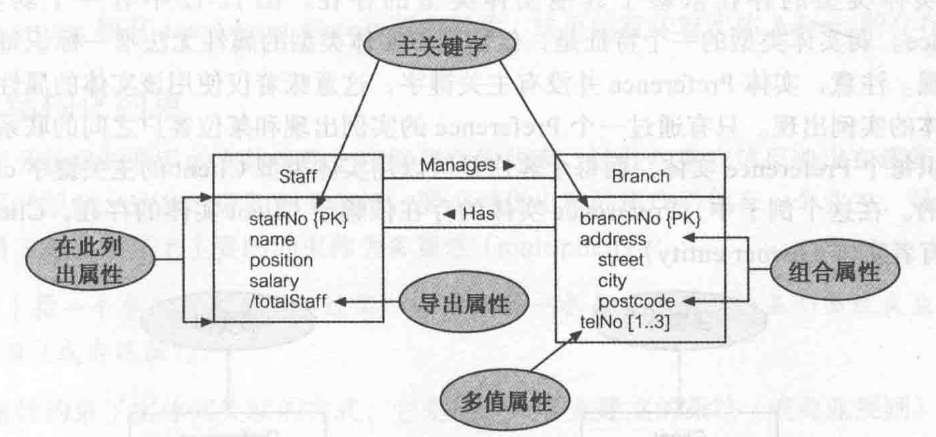UML中属性使用驼峰命名法。在属性组里，列出的第一个属性应该是主键，使用 ${PK}$ 标识。对于合成主键，使用 ${PPK}$ 标识。可替换键则使用 ${AK}$ 标识。对于一些复杂数据库系统，把每个实体类型的所有属性都列出来是不可能的。因此只需要列出每个实体的主键，这时可以省略 ${PK}$ 标识。组合属性需要在下方向右缩进列出子属性名。多值属性要明确属性取值个数的范围，可以使用 $*$ 表示 $1$ 个或者多个取值。导出属性需要在属性名前加上 $/$ 。

## 4. 强实体类型和弱实体类型

强实体类型 ( $Strong\ \ entity\ \ type$ ) 指存在不依赖于其他类型的实体类型。有时也被称为 ( $father$ ) 实体、所有者 ( $owner$ ) 实体或支配 ( $dominant$ ) 实体。 相反，弱实体类型 ( $weak\ \ entity\ \ type$ ) 指存在依赖于其他类型存在的类型。有时也被称为 ( $child$ ) 实体、依赖 ( $dependent$ ) 实体或者从属 ( $subordinate$ ) 实体。强实体类型的一个特征是可以使用该实体类型的主键唯一标识每个实体的实例出现，而弱实体类型不能。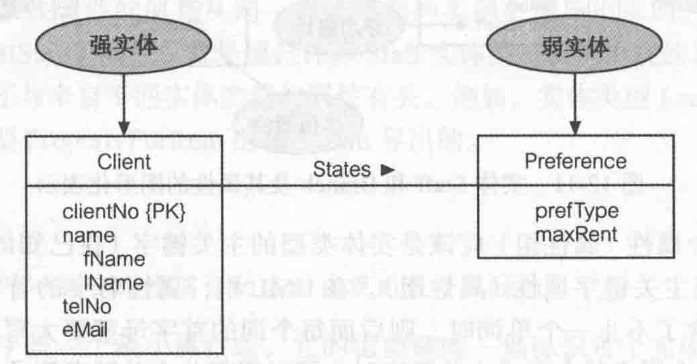## 5. 联系的属性在表示带有属性的联系时，使用虚线连接矩形和联系。一个联系如果拥有一个或者多个属性，那么该联系可能隐蔽着一个未标识的实体类型。

## 6. 结构化约束

多重性 ( $multiplicity$ ) 指一个参与实体类型通过某一联系与另一个参与实体类型的某个出现发生关联的出现数目，是联系上的主要约束。多重性约束了实体间关联的方式，是用户或企业建立的策略的一种表现方式。最常见的联系的度是二元的，二元又可以分为一对一、一对多和多对多。

### 6.1 一对一联系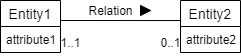$1..1$ 和 $0..1$ 是多重性的体现，分别表示一个 $Entity2$ 与一个 $Entity1$ 有联系，一个 $Entity1$ 可以与零至一个 $Entity2$ 有联系。

### 6.2 一对多联系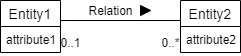### 6.3 多对多联系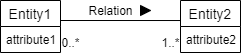### 6.4 复杂联系的多重性

复杂联系的多重性是指在一个 $n$ 元联系中，其他 $n - 1$ 个实体类型的值固定之后，另外一个实体类型可能参与联系的实例出现的个数。如一个三元联系的多重性表示当联系中的某两个参与实体类型的值确定后，剩下的那个参与实体的实例出现可能的个数。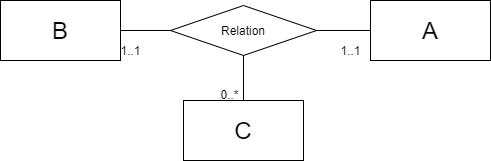$0..3$ $0$ 个到 $3$ 个实体出现
$0..*$ $0$ 个或者多个实体出现
$0$, $3$, $6-8$ $0$ 个、$3$ 个或者 $6$ 到 $8$ 个实体出现

### 6.5 基数约束和参与性约束

多重性由两个独立约束组成，基数 ( $cardinality$ ) 约束和参与性 ( $participation$ ) 约束。基数是在指定的联系类型中一个实体可能参与的联系出现的最大数目。一个二元联系的基数就是一对一、一对多和多对多。参与性说明所有实体出现是否都参与了联系。对于所有实体出现都参与的，称为强制 ( $mandatory$ ) 参与。否则，称为可选 ( $optional$ ) 参与。实体的参与性实际上就是联系的每一个参与实体的多重性范围的最小值，为 $0$ 时就是可选参与，为 $1$ 时就是强制参与。

## 7. ER模型的问题

创建ER模型时可能产生的问题称为连接陷阱 ( $connection\ \ trap$ )，通常是由于错误地理解了联系的意义引起的。

### 7.1 扇形陷阱

扇形陷阱 ( $fan\ \ trap$ ) 是指模型给出了两实体类型之间的一种联系，但是在某些实体出现之间存在着多条通路 ( $pathway$ )。当一个实体有两个或者更多的 $1:*$ 的联系时，就存在着扇形陷阱。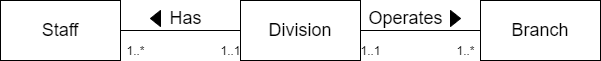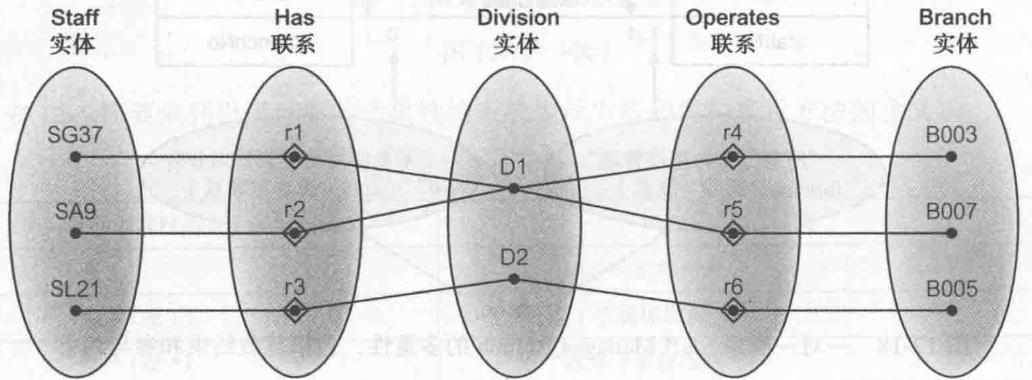上图为一个扇形陷阱的例子。在该图中，我们能很简单的找到每个 $Staff$ 的实体出现对应的 $Division$ ，但是无法找到其对应的 $Branch$ 。而为了消除该扇形陷阱，我们需要对该ER模型进行重构。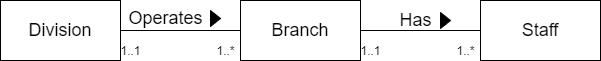### 7.2 断层陷阱

断层陷阱 ( $chasm\ \ trap$ ) 指模型表明某些实体类型之间存在联系，但在某些实体出现之间不存在通路的情况。当相关实体的路径上存在着一个或者多个多重性最小值为零的联系时，就会出现断层陷阱。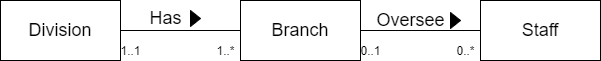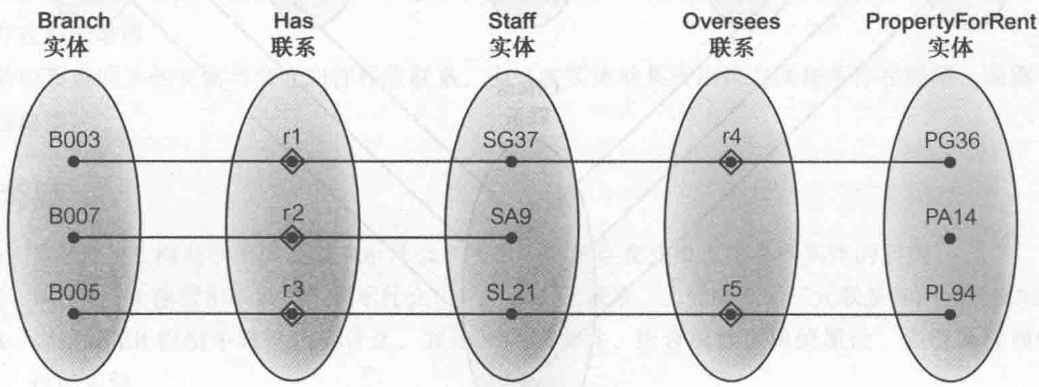显然对于 $PropertyForRent$ 中的实体出现 $PA14$ ，无法找到对应的 $Branch$ 的实体出现。解决方法是添加新联系。